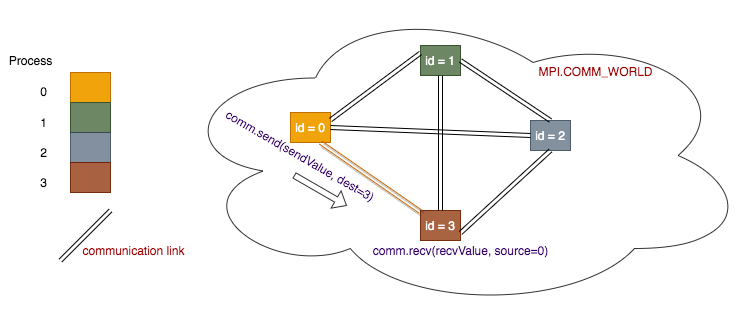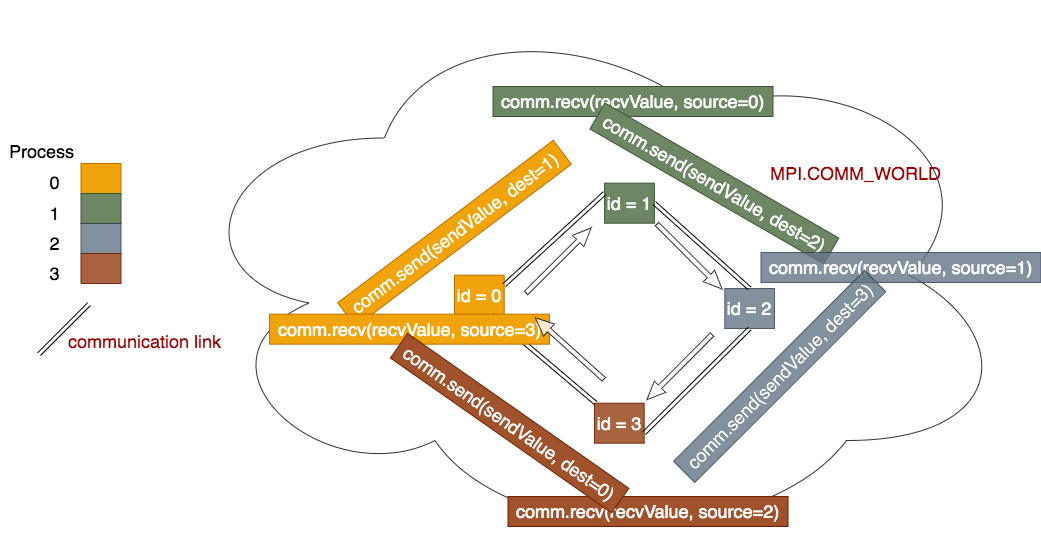# 2.2 Point to Point Communication¶

The fundamental basis of coordination between independent processes is point-to-point communication between processes through the communication links in the MPI.COMM_WORLD. The form of communication is called message passing, where one process sends data to another one, who in turn must receive it from the sender. This is illustrated as follows:Point-to-point communication is a way that pair of processors transmits the data between one another, one processor sending, and the other receiving. MPI provides Send() and Receive() functions that allow point-to-point communication taking place in a communicator. For general Python objects, use the lowercase send() and receive() functions instead.

Send(message, destination, tag)

- message:  numpy array containing the message
- destination:  rank of the receiving process
- tag:    message tag is a way to identify the type of a message (optional)

Recv(message, source, tag)
- message: numpy array containing the message
- source: rank of the sending process
- tag: message tag is a way to identify the type of a message


Note

The send() and recv() functions have identical form, except that the message parameter could be a standard Python type (e.g. int, string, float etc.). Be sure to use the uppercase form and numpy arrays whenever dealing with list-like data!

## Send and Receive Hello World¶

from mpi4py import MPI

def main():
comm = MPI.COMM_WORLD
id = comm.Get_rank()
numProcesses = comm.Get_size()

if id == 0:
hello_message = "Hello from master node!"
print("Process {0} (master) sending messages to workers: {1}".format(\
id, hello_message))

for i in range(1,numProcesses): #send to each worker process
comm.send(hello_message, dest=i)
else:
my_message = comm.recv(source=0)
print("Process {0} (worker) received message from master: {1}".format(\
id, my_message))

########## Run the main function
main()


This MPI program illustrates the use of send() and recv() functions. Notice that we use the lowercase version of the Send/Receive functions due to the fact that the message being sent is of type String.

The above program can be run using the following command:

python run.py ./sendReceive.py 4


## Integration - First Attempt¶

The code listing below illustrates how the integration example could be implemented with point-to-point communication:

from mpi4py import MPI
import math

#constants
n = 1048576 #number of trapezoids = 2**20

def f(x):
return math.sin(x)

def trapSum(my_a, my_b, my_n, h):
my_a*=h
my_b*=h
total = (f(my_a) + f(my_b))/2.0 #initial value
for i in range(1, my_n): #for each trapezoid
total += f(my_a+i*h)

def main():
comm = MPI.COMM_WORLD
id = comm.Get_rank()
numProcesses = comm.Get_size()

#desired range
a, b = 0, math.pi

#all processes: compute local variables
local_n  = n/numProcesses #num trapezoids per process
start  = id*local_n #starting trapeziod
end = (id+1)*local_n #ending trapezoid
if id == numProcesses-1: #in case processors don't divide things evenly
end = n
h = (b-a)/n #width of trapezoid (scaling factor)

#all processes: calculate local sum
my_sum = trapSum(start, end, local_n, h)

if id != 0: #if a worker process
comm.send(my_sum, dest=0) #send master the sum

else: #master process
results = [0.0]*numProcesses #generate master list to hold results
results = my_sum #places master's local sum in first element

for i in range(1,numProcesses):
other_sum = comm.recv(source=i) #get local sums from other processes
results[i] = other_sum #place in result array

print("Done receiving all messages")
print("Final sum is {0}".format(sum(results)))

########## Run the main function
main()


The trapSum() function computes and sums up a set of n trapezoids with a particular range. The first part of the main() function follows the SPMD pattern. Each process computes its local range of trapezoids and calls the trapSum() function to compute its local sum.

The latter part of the main() function follows the master-worker pattern. Each worker process sends its local sum to the master process. The master process generates a global array (called results), receives the local sum from each worker process, and stores the local sums in the results array. A final call to the sum() functon adds all the local sums together to produce the final result.

In later sections, we will see how to improve this example with other communication constructs. For now, ensure that you are comfortable with the workings of this program. You can execute it with the command:

python run.py ./integration.py 4


(multiple choice about why master worker pattern must be used, and why results cannot be shared)

## Exercise - Populate an Array¶

As an exercise, let’s use point-to-point communication to populate an array in parallel. The algorithm is as follows:

• Each process computes its local range of values, and then calls a function that generates an array of just those values.

• Each worker process sends its array to the master process.

• The master process generates a master array of the desired length, receives the local array from workers, and then populates the master array with the elements of the local arrays received.

The following program is a partially filled in solution, with the algorithm shown in comments.

from mpi4py import MPI
import numpy as np

#array size
N = 2000000

def populateArray(rank, nprocs):

#declare local variables:
nElems = #number of elemes to generate
start =  #starting element
end =  #end element
length = #length of array to generate

#generate empty array
local_array = np.empty(length)

#fill with desired values (start+1..end+1)
for i in range(length):
local_array[i] = #value at index i

return local_array

def main():
comm = MPI.COMM_WORLD
myId = comm.Get_rank()
numProcesses = comm.Get_size()

#SPMD pattern
local = populateArray(myId, numProcesses) #generate a local array

if (myId != 0):
#send local array to master

else:
#initialize global array, and fill with master's elements
global_array = np.empty(N)

#copy master's local array to
for i in range(len(local)):
global_array[i] = local[i]

#receive local arrays from each worker,
#and merge into global_array

#solution check:  the two sums should be equal
total = sum(global_array)
print(total)
print((N*(N+1)/2))

########## Run the main function
main()


Fill in the rest of the program, save as tryPopulateArray.py and test your program using the following commands:

python run.py ./tryPopulateArray.py 1
python run.py ./tryPopulateArray.py 2
python run.py ./tryPopulateArray.py 4


Remember, in order for a parallel program to be correct, it should return the same value with every run, and regardless of the number of processes chosen. Click the button below to see the solution.

## Ring of passed messages¶

Another pattern that appears in message passing programs is to use a ring of processes: messages get sent in this fashion:When we have 4 processes, the idea is that process 0 will send data to process 1, who will receive it from process 0 and then send it to process 2, who will receive it from process 1 and then send it to process 3, who will receive it from process 2 and then send it back around to process 0.

Program file: 07messagePassing5.py

from mpi4py import MPI

def main():
comm = MPI.COMM_WORLD
id = comm.Get_rank()            #number of the process running the code
numProcesses = comm.Get_size()  #total number of processes running
myHostName = MPI.Get_processor_name()  #machine name running the code

if numProcesses > 1 :

if id == 0:        # master
#generate a list with master id in it
sendList = [id]
# send to the first worker
comm.send(sendList, dest=id+1)
print("Master Process {} of {} on {} sent {}"\
.format(id, numProcesses, myHostName, sendList))
# receive from the last worker
print("Master Process {} of {} on {} received {}"\
else :
# worker: receive from any source
# add this worker's id to the list and send along to next worker,
# or send to the master if the last worker
comm.send(sendList, dest=(id+1) % numProcesses)

print("Worker Process {} of {} on {} received {} and sent {}"\

else :
print("Please run this program with the number of processes \
greater than 1")

########## Run the main function
main()


Exercise:

Run the above program varying the number of processes for N = 1 through 8/

python run.py ./07messagePassing3.py N


Compare the results from running the example to the code above. Make sure that you can trace how the code generates the output that you see.

Exercise: